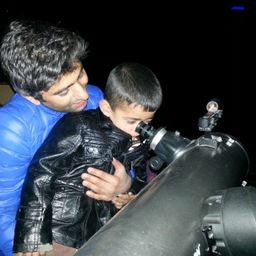Gravitation

### Discussion

You must be signed in to discuss.
##### Top Physics 101 Mechanics Educators##### Christina K.

Rutgers, The State University of New Jersey##### Jared E.

University of WinnipegLectures

Join Bootcamp

### Video Transcript

well speed off meter stick relative to the earth's surface. We is equal to 0.900 times the speed off light. Uh, let's do party in party. Let's find out Meter sticks. Lent is major by the Observer. A crest with respect to the earth's surface. Now using l is equal to not times square root off one minus e. We skated by the basis were one minus. We squared, divided by C square knot. Let's look in the values since we have a meter stick. So at Monte is one meter into a squared Rudolph one minus Well, we is 0.900 times the speed of light square divided by C Square and therefore L is equal to one meter. Multiply by screen Rudolph a 0.19 and it's equal to 0.4 36 leaders. Okay, now let's to part B. Well, if the observer starts running toward a meter stick by observer, What server start? Uh, running. Running the word. Two word. Um, the meter stick the major steep. Then, uh, the speed of the meter stick for the observer will increase on so we will increase. We will increase speed with Linc Res and therefore the meter stick will contract more. So the meter the meter stick steak will contract will contract more. So if the observer starts running toward the meter, stick the speed of the meter, stick for the observer will increase and therefore the meter state will contract more.#### Topics

Gravitation

##### Top Physics 101 Mechanics Educators##### Christina K.

Rutgers, The State University of New Jersey##### Jared E.

University of WinnipegLectures

Join Bootcamp